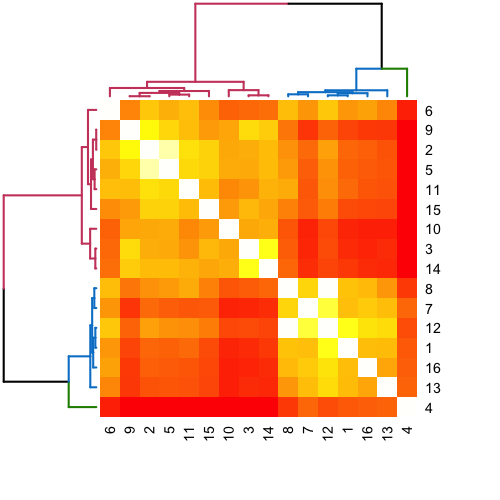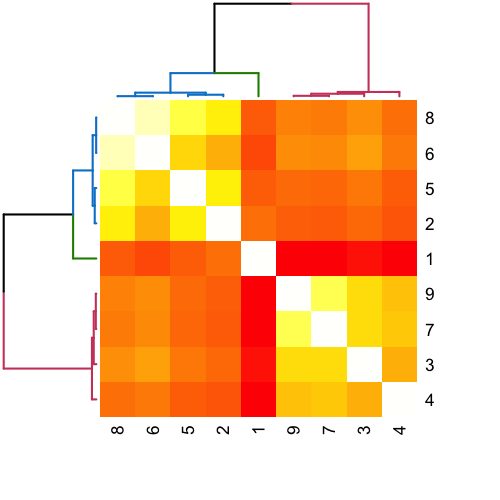# ccc/hsbm (Friendster)

CEP & his team
Department of Applied Mathematics and Statistics
Johns Hopkins University

### Data

The data is originally from: http://snap.stanford.edu/data/com-Friendster.html

J. Yang and J. Leskovec. “Defining and Evaluating Network Communities based on Ground-truth”. ICDM, 2012.

### Experiment

1. Take the largest connected component (“lcc”) of $$G$$, denoted $$G'=(V',E')$$.
(turns out that $$G = G'$$: $$order(G') = |V'| = 65,608,366$$ nodes and $$size(G') = |E'|=1,806,067,135$$ edges.)
2. Embed $$G'$$ into $$R^{d=50}$$ using adjacency spectral embedding, denoted $$\hat{X}$$.
3. Find an elbow: $$\hat{d} = 14$$.
4. Cluster $$\hat{X} \in \mathbb{R}^{14}$$ into $$\hat{R}=16$$ clusters determined by average silhouette width criterion, denote each subgraph as $$\hat{H}_r$$.

Summary of the clusters:

cluster subg.order subg.size lcc.order lcc.size
1 3173696 72340821 3108471 72334735
2 2848386 9809441 1599429 9779706
3 8576519 69283994 7870961 69245017
4 1514469 57410892 1330725 57371638
5 3771122 35569898 3729532 35567654
6 2478497 11371576 1319927 11347569
7 1557016 19108803 1416238 19079683
8 3901613 59921089 3407165 59836037
9 4376262 27046756 4113274 27030566
10 9140083 54467095 7558797 54369954
11 4529703 44110968 4278925 44099897
12 3267543 63617581 3164782 63612517
13 1167628 13690178 1115613 13685395
14 5311510 31262924 4624917 31205512
15 7194615 134588427 7189683 134588231
16 2799704 70325838 2758483 70323822
#     cluster        subg.order        subg.size           lcc.order
#  Min.   : 1.00   Min.   :1167628   Min.   :  9809441   Min.   :1115613
#  1st Qu.: 4.75   1st Qu.:2719402   1st Qu.: 25062268   1st Qu.:1553631
#  Median : 8.50   Median :3519332   Median : 49289032   Median :3285974
#  Mean   : 8.50   Mean   :4100523   Mean   : 48370393   Mean   :3661683
#  3rd Qu.:12.25   3rd Qu.:4725155   3rd Qu.: 65034184   3rd Qu.:4365423
#  Max.   :16.00   Max.   :9140083   Max.   :134588427   Max.   :7870961
#     lcc.size
#  Min.   :  9779706
#  1st Qu.: 25042845
#  Median : 49234926
#  Mean   : 48342371
#  3rd Qu.: 65020642
#  Max.   :134588231

5. Reembed $$\hat{H}_r$$ into $$\mathbb{R}^{d=100}$$ using adjacency spectral embedding, denoted $$\hat{X}_r$$.

6. Calculate dissimilarity measures among $$\hat{X}_r$$ using a kernel method, denoted $$\hat{S}$$.

7. Cluster $$\hat{S}$$ to estimate $$\hat{B}$$ and $$\hat{\rho}$$.prepared by [email protected] on Mon Mar 9 16:57:26 2015